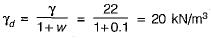Courses

# Test: Properties Of Soil - 1

## 10 Questions MCQ Test Topicwise Question Bank for GATE Civil Engineering | Test: Properties Of Soil - 1

Description
This mock test of Test: Properties Of Soil - 1 for Civil Engineering (CE) helps you for every Civil Engineering (CE) entrance exam. This contains 10 Multiple Choice Questions for Civil Engineering (CE) Test: Properties Of Soil - 1 (mcq) to study with solutions a complete question bank. The solved questions answers in this Test: Properties Of Soil - 1 quiz give you a good mix of easy questions and tough questions. Civil Engineering (CE) students definitely take this Test: Properties Of Soil - 1 exercise for a better result in the exam. You can find other Test: Properties Of Soil - 1 extra questions, long questions & short questions for Civil Engineering (CE) on EduRev as well by searching above.
QUESTION: 1

### For a sandy soil with soil grains spherical in shape and uniform in size, what is the theoretical void ratio?

Solution: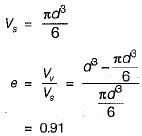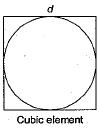QUESTION: 2

### The laboratory tests on a sample yielded the following results: Plasticity index : 32% Liquidity index 0.15 Activity number 1.58 Which of the following inferences can be drawn? 1. The soil is very stiff. 2. The soil is medium soft. 3. The soil is highly plastic, 4. The soil is medium plastic. 5. The soil is active. Select the correct answer using the codes given below:

Solution: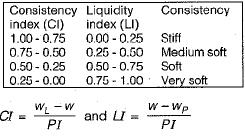where
w is natural water content
wL is liquid limit
wp is plastic limit
PI is plasticity index
Cl + LI = 1.00
If Cl > 1 or LI < 0, then soil is very stiff.
If Cl < 0 or LI > 1 soil behaves as liquid.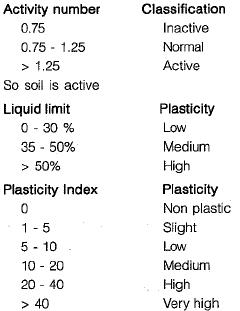So soil is high plastic

QUESTION: 3

### A clay sample has a void ratio 0.54 in dry state. The specific gravity of soil solids is 2.7, What is the shrinkage limit of the soil?

Solution:

At shrinkage limit, the soil remains fully saturated.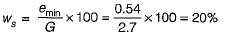QUESTION: 4

Match List-I (Unit/Test) with List-ll (Purpose) and select the correct answer using the codes given below the lists:
List-I
A. Casagrande’s apparatus
B. Hydrometer
C. Plate load test
D. Oedometer
List-ll
1. Determination of grain size distribution
2. Consolidation characteristics
3. Determination of consistency limits
4. Determination of safe bearing capacity of soil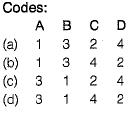Solution:
QUESTION: 5

Consistency as applied to cohesive soils is an indicator of its

Solution:

Consistency of soil refers to the resistance offered by it against forces that tend to deform or rupture the soil aggregate. It is related to strength.

QUESTION: 6

The plasticity index and the percentage of grain size finer than 2 microns of a clay sample are 25 and 15, respectively. Its activity ratio is

Solution:

Activity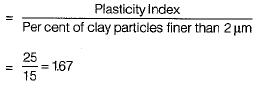As activity is more than 1.25 so it is active soil.

QUESTION: 7

The natural void ratio of a sand sample is 0.6 and its density index is 0.6. If its void ratio in the loosest state is 0.9, then the void ratio in the densest state will be

Solution: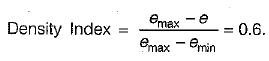Void ratio in loosest state, emax = 0.9
Void ratio in natural state, e = 0.6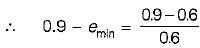emin = 0.9 - 0.5 = 0.4

QUESTION: 8

Which one of the following correctly represents the dry unit weight of a soil sample which has a bulk unit weight of γat a moisture content of W%?

Solution: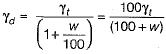QUESTION: 9

A soil has a liquid limit of 40% and plasticity index of 20%. The plastic limit of the soil will be

Solution:

Plastic limit, WP = WL - PI = 40 - 20 = 20%

QUESTION: 10

A soil has bulk density of 22 kN/m3 and water content 10%. The dry density is

Solution: